Worksheet Solution: Ways of Multiply and Divide

# Worksheet Solution: Ways of Multiply and Divide Notes | Study Mathematics for Class 5: NCERT - Class 5

## Document Description: Worksheet Solution: Ways of Multiply and Divide for Class 5 2022 is part of Mathematics for Mathematics for Class 5: NCERT preparation. The notes and questions for Worksheet Solution: Ways of Multiply and Divide have been prepared according to the Class 5 exam syllabus. Information about Worksheet Solution: Ways of Multiply and Divide covers topics like and Worksheet Solution: Ways of Multiply and Divide Example, for Class 5 2022 Exam. Find important definitions, questions, notes, meanings, examples, exercises and tests below for Worksheet Solution: Ways of Multiply and Divide.

Introduction of Worksheet Solution: Ways of Multiply and Divide in English is available as part of our Mathematics for Class 5: NCERT for Class 5 & Worksheet Solution: Ways of Multiply and Divide in Hindi for Mathematics for Class 5: NCERT course. Download more important topics related with Mathematics, notes, lectures and mock test series for Class 5 Exam by signing up for free. Class 5: Worksheet Solution: Ways of Multiply and Divide Notes | Study Mathematics for Class 5: NCERT - Class 5
 1 Crore+ students have signed up on EduRev. Have you?

Q.1. Kishan drinks 6 glasses of water every day.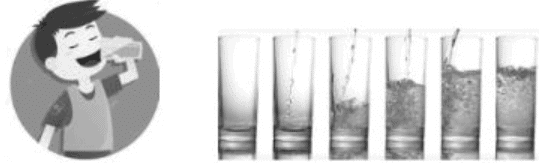How many glasses of water will he drink m one week?
Ans.
In one day, the amount of water he drinks is = 6 glasses.
In one week total days = 7.
So amount of water drunk by Kishan = 6 glasses x 7 days of week = 42 glasses of water.

Q.2. Kishan drinks 6 glasses of water every day.How many glasses of water will he drink in 1 month?
Ans.
In one day, the amount of water he drinks is = 6 glasses
In one month, total days = 30 days
so. total water drunk by him = 6 glass x 30 days of month = 180 glass of water.

Q.3. Kishan drinks 6 glasses of water every day.How many glasses of water will he drink in one year?
Ans.
In one day the amount of water he drinks is = 6 glasses
In one year, total days = 365 days
So total water drunk by him in 1 year = 6 x 365 = 2190 glasses of water.

Q.4. Kishan drinks 6 glasses of water every day.How many glasses of water will he drink in 1440 hours?
Ans. In one day, the amount of water he drinks is = 6 glasses
In one day, total hours = 24 hours
In 1440 hours number of days = 1440/24 = 60 days.
Therefore, total amount of water drunk by him = 60 x 6 = 360 glass.

Q.5. Kishan drinks 6 glasses of water every day.If there are 15 members m his family, then how many glass of water will the family drink in 1 year?
Ans. In one day, the amount of water he drinks is = 6 glasses
In one year, total days = 365 days
So total water drunk by him in 1 year = 6 x 365 = 2190 glasses of water.
Therefore, amount of water drunk by his family in one year is = 2190 x 15 = 32850 glasses of water.

Q.6. Multiply 357 by 23?
Ans.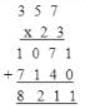Q.7. Lakshya works on a garden and gets paid Rs 98 per day
How much will he earn if he works for 28 days?
Ans.
The amount he gets for 1 day = Rs 98
The amount he gets for 28 days = Rs 98 x 28 days = Rs 2,744

Q.8. Lakshya works on a garden and gets paid Rs 98 per day
How much will he earn for working 97 days?
Ans. The amount he gets for working 97 days
= Rs 98 x 97 days
= Rs 9506

Q.9. Divide the following:
(a) 12111 / 11
(b) 96888 / 22
(c) 6548 / 4
(d) 65484 / 9
Ans.
(a) 1101
(b) 4404
(C) 1637
(d) 7276

Q.10. 875 children are going for a picnic with school min bus. Each bus can accommodate 25 children. How many buses will they need in total?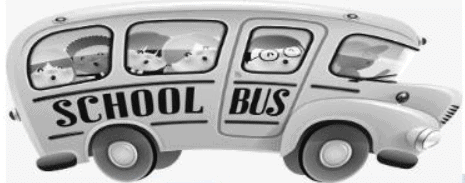Ans.
Total children going for picnic = 875
Total children a bus can accommodate = 25
Therefore. Total number of buses required = 875/25 = 35 buses.

Q.11. 875 children are going for a picnic with school min bus. Each bus can accommodate 25 children. How many buses will they need in total if 50 more teachers are going with them?Ans. Total children going for picnic = 875
Total teachers going for picnic = 50
Total number of people going for the picnic = 875 + 50 = 925
Total people a bus can accommodate = 25
Therefore Total number of buses required = 925/25 = 37 buses.

Q.12. 875 children are going for a picnic with school min bus. Each bus can accommodate 25 children. How many buses will they need in total? How much fare will be collected in total if the travelling charge for bus is Rs 97 per person?Ans.
Total children going for picnic = 875
Total children a bus can accommodate = 25
Therefore Total number of buses required = 875/25 = 35 buses
Fare given per person = Rs 97
Total fare given by 875 children = 97 x 875 = Rs 84875

Q.13. Sameer has arranged for a party with 26 friends. He has collected the total contribution of Rs 15903. How much money did each person contribute?
Ans.
Total amount collected for the party = Rs 15903
Total number of people who contributed for party = 26 + 1 = 27
Total amount contributed by each person = Rs 15905 /27 = Rs 589

Q.14. Sameer has arranged for a party with 26 friends. He has collected the total contribution of Rs 15903. After party, Rs 4293 was left. How much amount should he return to every person?
Ans.
Total amount left at the end of party = Rs 4293
Total number of people in party = 26 + 1 = 27
Total money to be returned to every person = Rs 4293/27 = Rs 159

Q.15. Manu has Rs 2000 to buy petrol. If price of petrol is Rs 51 per liter. How many liters of petrol will he get?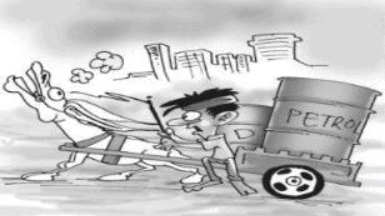Ans.
Total money with Manu = Rs 2000
Price of petrol = Rs 51 per liter.
Total liters of petrol he can buy = 2000/51 = 39 liters

Q.16. Manu has Rs 2000 to buy petrol. If price of petrol is Rs 51 per liter. How many liters of petrol will he get? How much money will be left with him?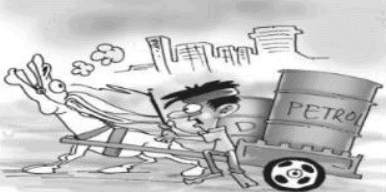Ans.
Total money with Manu = Rs 2000
Price of petrol = Rs 51 per liter
Total liters of petrol he can buy = 2000/51 = 39 (remainder 11? = 39 liters
Total money left with him = 2000 - (39 x 51)
= 2000 - 1989
= 11.
Therefore, total money left with him = remainder = Rs 11

Q.17. If a car moves 17 km in one liter, how long will it go in 351 liters of petrol?
Ans.
In one liter, distance moved = 17 km
Total distance covered m 351 liters of petrol = 17 x 351
= 5967 km

Q.18. If a car moves 19 km in 1 liter of deisel, how many liters of deisel will be required to move 2,356 km?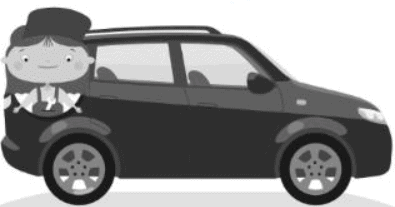Ans.
Distance covered by car in 1 liter of deisel = 19km
Or, Deisel required to move 19 km = 1 liter
Deisel required to move 2,356 km = 2,356/19 =124 liters

Q.19. How many pencils will remain with Sumit if he distributes 459 pencils among his 18 classmates?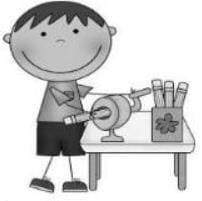Ans.
459/18 = 25 (remainder = 9)
Therefore, number of pencils remaining with Sumit = remainder
= 9 pencils.

Q.20. Sumit has 4531 pencils and he gives these pencils equally to his 21 friends. How many pencils will every friend get if they are distributed equally among 21 friends?Ans.
Total number of pencils = 4531
Total friends = 21
Total pencils even.- friend gets = 4531/21 = 215 pencils

The document Worksheet Solution: Ways of Multiply and Divide Notes | Study Mathematics for Class 5: NCERT - Class 5 is a part of the Class 5 Course Mathematics for Class 5: NCERT.
All you need of Class 5 at this link: Class 5

## Mathematics for Class 5: NCERT

33 videos|58 docs|39 tests
 Use Code STAYHOME200 and get INR 200 additional OFF

## Mathematics for Class 5: NCERT

33 videos|58 docs|39 tests

Track your progress, build streaks, highlight & save important lessons and more!

,

,

,

,

,

,

,

,

,

,

,

,

,

,

,

,

,

,

,

,

,

;